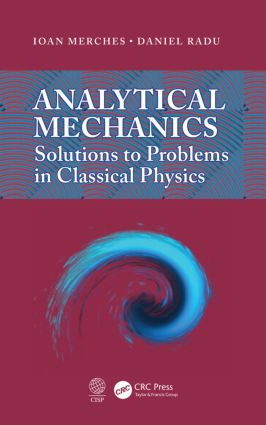Analytical Mechanics

Solutions to Problems in Classical Physics, 1st Edition

CRC Press

456 pages | 122 B/W Illus.

Purchasing Options:\$ = USD
Hardback: 9781482239393
pub: 2014-08-26
SAVE ~\$14.19
\$70.95
\$56.76
x
eBook (VitalSource) : 9780429068614
pub: 2014-08-26
from \$35.48

FREE Standard Shipping!

Description

Giving students a thorough grounding in basic problems and their solutions, Analytical Mechanics: Solutions to Problems in Classical Physics presents a short theoretical description of the principles and methods of analytical mechanics, followed by solved problems. The authors thoroughly discuss solutions to the problems by taking a comprehensive approach to explore the methods of investigation. They carefully perform the calculations step by step, graphically displaying some solutions via Mathematica® 4.0.

This collection of solved problems gives students experience in applying theory (Lagrangian and Hamiltonian formalisms for discrete and continuous systems, Hamilton-Jacobi method, variational calculus, theory of stability, and more) to problems in classical physics. The authors develop some theoretical subjects, so that students can follow solutions to the problems without appealing to other reference sources. This has been done for both discrete and continuous physical systems or, in analytical terms, systems with finite and infinite degrees of freedom. The authors also highlight the basics of vector algebra and vector analysis, in Appendix B. They thoroughly develop and discuss notions like gradient, divergence, curl, and tensor, together with their physical applications.

There are many excellent textbooks dedicated to applied analytical mechanics for both students and their instructors, but this one takes an unusual approach, with a thorough analysis of solutions to the problems and an appropriate choice of applications in various branches of physics. It lays out the similarities and differences between various analytical approaches, and their specific efficiency.

Fundamentals of Analytical Mechanics

Constraints

Classification Criteria for Constraints

The Fundamental Dynamical Problem for a Constrained Particle

System of Particles Subject to Constraints

Lagrange Equations of the First Kind

Elementary Displacements

Generalities

Real, Possible and Virtual Displacements

Virtual Work and Connected Principles

Principle of Virtual Work

Principle of Virtual Velocities

Torricelli’s Principle

Principles of Analytical Mechanics

D’alembert’s Principle

Configuration Space

Generalized Forces

Hamilton’s Principle

The Simple Pendulum Problem

Classical (Newtonian) Formalism

Lagrange Equations of the first Kind Approach

Lagrange Equations of the Second Kind Approach

Hamilton’s Canonical Equations Approach

Hamilton-Jacobi Method

Action-Angle Variables Formalism

Problems Solved by Means of the Principle of Virtual Work

Problems of Variational Calculus

Elements of Variational Calculus

Functionals. Functional Derivative

Extrema of Functionals

Problems whose solutions demand elements of variational calculus

Brachistochrone problem

Catenary problem

Isoperimetric problem

Surface of revolution of minimum area

Geodesics of a Riemannian manifold

Problems Solved by Means of the Lagrangian Formalism

Atwood machine

Double Atwood Machine

Pendulum with Horizontally Oscillating Point of Suspension

Problem of Two Identical Coupled Pendulums

Problem of Two Different Coupled Pendulums

Problem of Three Identical Coupled Pendulums

Problem of Double Gravitational Pendulum

Problems of Equilibrium and Small Oscillations

Problems Solved By Means of the Hamiltonian Formalism

Problems of Continuous Systems

A. Problems of Classical Electrodynamics

B. Problems of Fluid Mechanics

C. Problems of Magnetofluid Dynamics and Quantum Mechanics

APPENDICES

REFERENCES